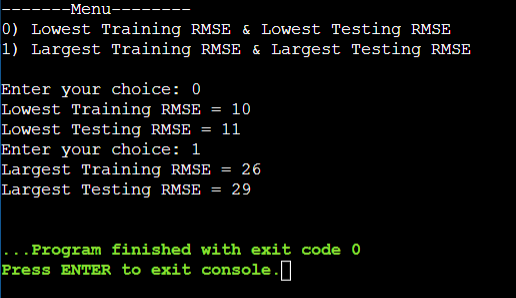# This is a plain c language. I need help with coming up with codes

## Question

Printing a general output along with a customized message to standard output. The general output shows the two values for each model. The customized output is selected by the user. The program will ask the user to choose between two options: (0): Show the model numbers with the lowest training RMSE and the lowest testing RMSE; (1) Show the model numbers with the largest training RMSE and the largest testing RMSE. If the user inputs a different value, the program will execute all its tasks but no customized message will be shown.

Important: The model with the largest/lowest training RMSE is not necessarily the same model with the largest/lowest testing RMSE.
How would I write codes so that it prints a general output along with a customized message to standard output?

//lowest
if ( rmse1 <= rmse2 )
if ( rmse1 <= rmse3 )
best = 1;
else
best = 3;
else if ( rmse2 <= rmse3 )
best = 2;
else
best = 3;
end
//higest
if ( rmse1 >= rmse2 )
if ( rmse1 >= rmse3 )
worst = 1;
else
worst = 3;
else if ( rmse2 >= rmse3 )
worst = 2;
else
worst = 3;
end

## Summary

A customized prompt message will be shown to the user, to choose between two available options. If the user opts for 0, then the lowest training rmse and lowest testing rmse will be found and displayed as output.

Otherwise, the largest training rmse and largest testing rmse will be displayed as output. For this, we have implemented a model structure. We have to print the general code.

## Explanation

Let’s consider three models as shown below with the RMSE train and RMSE test.
Model1 -> RMSE train=10, RMSE test=11
Model2 -> RMSE train=26, RMSE test=29
Model3 -> RMSE train=12, RMSE test=14
The lowest RMSE train = 10.
The lowest RMSE test = 11.
The largest RMSE train = 26.
The largest RMSE test = 29.

We have created the structure model with the members rmseTrain and rmseTest. Then, in the main method, we have declared three model variables and initialized their members. Then, the user will prompt to show the available options. If the user chooses 0, then from the three models, we will print the lowest train and test. And if the user chooses 1, then from the three models, we will print the largest train and test.

## Code

#include <stdio.h>
/* to implement model structure */
struct model{
/* members */
int rmse_train, rmse_test;

};
/* driver code */
int main()
{
/* three models */
struct model mdl1; mdl1.rmse_train=10; mdl1.rmse_test=11;
struct model mdl2; mdl2.rmse_train=26; mdl2.rmse_test=29;
struct model mdl3; mdl3.rmse_train=12; mdl3.rmse_test=14;
printf("-------Menu--------\n0) Lowest Training RMSE & Lowest Testing RMSE\n");
printf("1) Largest Training RMSE & Largest Testing RMSE\n\n");
for(int i=0;i<2;i++){
int ch;
scanf("%d",&ch);
if(ch==0){
/* to find the lowest rmse train */
if(mdl1.rmse_train<mdl2.rmse_train && mdl1.rmse_train<mdl3.rmse_train)
printf("Lowest Training RMSE = %d\n",mdl1.rmse_train);
else if(mdl2.rmse_train<mdl1.rmse_train && mdl2.rmse_train<mdl3.rmse_train)
printf("Lowest Training RMSE = %d\n",mdl2.rmse_train);
else if(mdl3.rmse_train<mdl2.rmse_train && mdl3.rmse_train<mdl1.rmse_train)
printf("Lowest Training RMSE = %d\n",mdl3.rmse_train);
/* to find the lowest rmse test */
if(mdl1.rmse_test<mdl2.rmse_test && mdl1.rmse_test<mdl3.rmse_test)
printf("Lowest Testing RMSE = %d\n",mdl1.rmse_test);
else if(mdl2.rmse_test<mdl1.rmse_test && mdl2.rmse_test<mdl3.rmse_test)
printf("Lowest Testing RMSE = %d\n",mdl2.rmse_test);
else if(mdl3.rmse_test<mdl2.rmse_test && mdl3.rmse_test<mdl1.rmse_test)
printf("Lowest Testing RMSE = %d\n",mdl3.rmse_test);
}
else if(ch==1){
/* to find the largest rmse train */
if(mdl1.rmse_train>mdl2.rmse_train && mdl1.rmse_train>mdl3.rmse_train)
printf("Largest Training RMSE = %d\n",mdl1.rmse_train);
else if(mdl2.rmse_train>mdl1.rmse_train && mdl2.rmse_train>mdl3.rmse_train)
printf("Largest Training RMSE = %d\n",mdl2.rmse_train);
else if(mdl3.rmse_train>mdl2.rmse_train && mdl3.rmse_train>mdl1.rmse_train)
printf("Largest Training RMSE = %d\n",mdl3.rmse_train);
/* to find the largest rmse test */
if(mdl1.rmse_test>mdl2.rmse_test && mdl1.rmse_test>mdl3.rmse_test)
printf("Largest Testing RMSE = %d\n",mdl1.rmse_test);
else if(mdl2.rmse_test>mdl1.rmse_test && mdl2.rmse_test>mdl3.rmse_test)
printf("Largest Testing RMSE = %d\n",mdl2.rmse_test);
else if(mdl3.rmse_test>mdl2.rmse_test && mdl3.rmse_test>mdl1.rmse_test)
printf("Largest Testing RMSE = %d\n",mdl3.rmse_test);
}
}
}


## Output